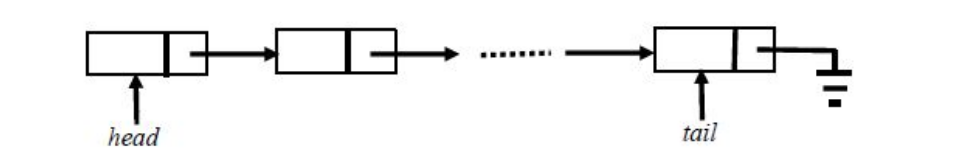# GATE | GATE CS 2018 | Question 11

A queue is implemented using a non-circular singly linked list. The queue has a head pointer and a tail pointer, as shown in the figure. Let n denote the number of nodes in the queue. Let ‘enqueue’ be implemented by inserting a new node at the head, and ‘dequeue’ be implemented by deletion of a node from the tail.Which one of the following is the time complexity of the most time-efficient implementation of ‘enqueue’ and ‘dequeue, respectively, for this data structure?
(A) Θ(1), Θ(1)
(B) Θ(1), Θ(n)
(C) Θ(n), Θ(1)
(D) Θ(n), Θ(n)

Explanation: For Enqueue operation, performs in constant amount of time (i.e., Θ(1)), because it modifies only two pointers, i.e.,

```Create a Node P.
P-->Data = Data
```

For Dequeue operation, we need address of second last node of single linked list to make NULL of its next pointer. Since we can not access its previous node in singly linked list, so need to traverse entire linked list to get second last node of linked list, i.e.,

```temp = head;
While( temp-Next-->Next != NULL){
temp = temp-Next;
}
temp-->next = NULL;
Tail = temp;
```

Since, we are traversing entire linked for each Dequeue, so time complexity will be Θ(n).

Option (B) is correct.

Quiz of this Question

My Personal Notes arrow_drop_up
Article Tags :

1

Please write to us at contribute@geeksforgeeks.org to report any issue with the above content.